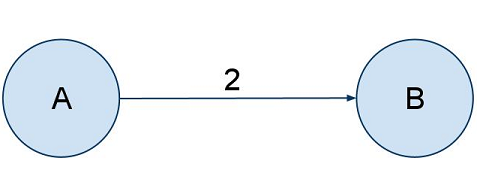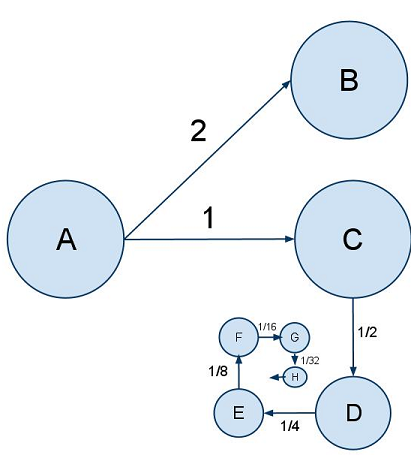# The Harmonic Series

### February 17, 2011 12:00

All of my posts up to this point have been directly programming related, but I thought I'd take a little deviation from those posts and propose a bit of a different topic for this post. Why not move out of the realm of implementation and just discuss ideas?

I am currently taking an algorithm analysis class in which we discuss current algorithms, discuss their implementations, and analyze algorithms' time-efficiency. While on the subject of graphs, we began talking about Dijkstra's algorithm which calculates the shortest path between vertices in a graph. While already being very familiar with the idea, I started to do a little thinking of my own and ended up with a problem which entertained me for quite awhile. Let's take a look at it.

Suppose we have two vertices, A and B, connected by an edge of length 2If we were to start traveling along this edge at 1 unit per second, we would reach vertex B after 2 seconds. Very basic.

Now let's imagine another case. We have a graph with three vertices; A, B, and C. We have an edge from A to B of length 2 and another edge from A to C of length 1.If we were to start one signal (We will call signal X) from A to B and another from A to C (Signal Y) at the exact same moment, Y will reach vertex C in just 1 second while it will take another second for X to reach B.

Now suppose we have the following case.We have the same path to B, but after vertex C, there is an infinite set of vertices connected by an edge of length half that of the previous. So, we have lengths of 1, 1/2, 1/4, 1/8, and so on. Basic math tells us that the limit of this series is 2. But interestingly, it never reaches 2. It will approach 2 and become infinitely close, but will never get to 2. So, we'll perform the same thing we did with the previous test. We start two signals at A (X and Y). X travels towards B at a rate of 1 unit per second while Y travels towards C at the same speed. After signal Y reaches C, it continues on its way through D.

Simple enough. After 1 second, X is halfway between A and B. Y is at C. After 1.5 seconds, signal X is three-quarters of the way to B and signal Y is at D. And so on. But think about it; after two seconds, signal X has made it all the way to B. Where is signal Y? Did it end its path? Where was signal Y after 1.999 seconds? At the 2 second mark? Does it simply cease to exist? If it ceases to exist, doesn't that imply that it reached some kind of end? If not, why not?

A very abstract problem, but still fun to think about. Enjoy!

## Got something to say? Tell me!

Name*

Homepage

Comment*

Feb.26.2011
17:42

critical

does this mean there is an infinite amount of time between seconds?